# Scalar facts for kids

Kids Encyclopedia Facts

Scalars are simple numbers. They are used for measuring things. Many things can be measured, and the measure can be explained by only giving the number.

Suppose we are measuring a rod. We could give the measured length as 2 metres or 3 cm—depending on the length of the rod under observation. Here, only the figure 2 or 3 along with the unit of measure is enough to transmit information about the rod.

Other examples of scalars are:

In other words, mass is measured by scalars, just like distances are measured by scalars.

In mathematics, scalars are distinguished from more complex mathematical objects such as vectors and matrices in that they consist of only one number. A scalar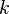$k$ can also be multiplied to a vector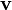$\mathbf{v}$ or a matrix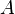$A$, resulting in the vector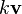$k\mathbf{v}$ and the matrix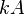$kA$, respectively. For vectors, scalar multiplication produces a new vector of different length in the same or opposite direction of the original vector.

## Related pagesScalar Facts for Kids. Kiddle Encyclopedia.